Latest Banking jobs   »   Quantitative Aptitude Quiz For IBPS PO...

# Quantitative Aptitude Quiz For IBPS PO Mains 2022-22nd January

Directions (1-2): There are three toys which are in cylindrical, conical and spherical shape. Height of all the toys is same. Ratio between the height of cylinder to radius of cylinder is 4 : 3.

Q1. Find the ratio between total surface area of cylinder to that of sphere.
(a) 17 : 15
(b) 21 : 16
(c) 28 : 23
(d) 21 : 8
(e) 15 : 4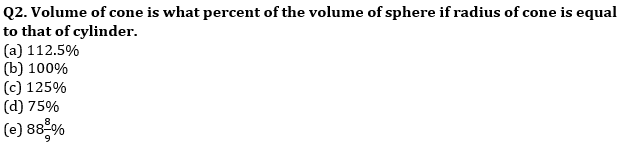Q3. P, Q and R have 120 mangoes in total. P give 37.5% of his mangoes to Q and R such that now he has equal number of mangoes as Q and R together have. After that Q give same % of mangoes to R, after which Q and R have equal number of mangoes. How many mangoes Q gives to R.
(a) 18
(b) 24
(c) 12
(d) 30
(e) 6

Directions (4-8): The following table shows different vessels, type of their shapes and different dimensions of these vessels.
(Note: Some values are missing, you need to calculate those values if required.)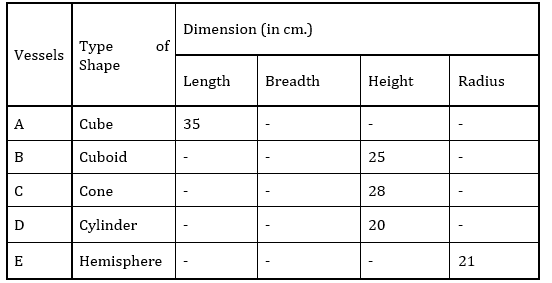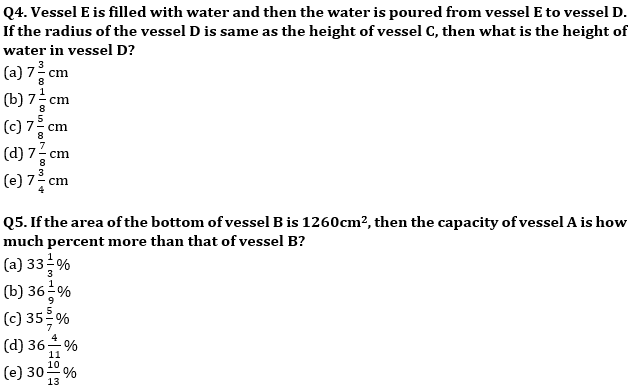Q6. What the ratio of lateral surface areas of vessel C and vessel E if the ratio of radius and height of vessel C is 3 : 4?
(a) 5 : 6
(b) 6 : 5
(c) 3 : 5
(d) 5 : 3
(e) 2 : 3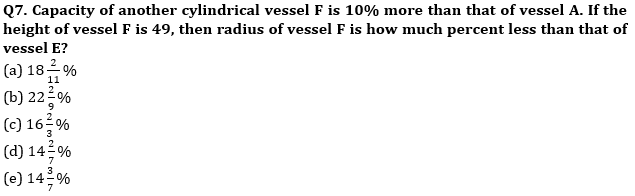Q8. Vessel D needs to be painted on the lateral surface while vessel A needs to be painted on lateral surface as well as on the bottom. What will be the total expenditure of painting these vessels if the cost of painting is Rs.0.2/cm² if the radius of the vessel D is same as the height of vessel C?
(a) Rs.1919
(b) Rs.1939
(c) Rs.1909
(d) Rs.1929
(e) Rs. 2019

Q9. Average of five two digit numbers, in which 2 numbers say A and B are reverse of each other, is 25.4. If all three numbers except A and B multiplied by (–2) then the average of five numbers decreased by 43.2. What could be the greatest value among A and B.
(a) 32
(b) 23
(c) 50
(d) 41
(e) 14

Q10. Two liquid containing (X and Y) mixture mixed, in the ratio 2 : 3. X contain 40% sugar in water, while Y contain alcohol in water. After adding 20 gram sugar in the final mixture ratio of Sugar, alcohol and water becomes 8 : 5 : 16. Given that all ratio is calculate on weight. Find the weight alcohol in mixture ‘Y’.
(a) 25 grams
(b) 50 grams
(c) 60 grams
(d) 80 grams
(e) 75 grams

Solutions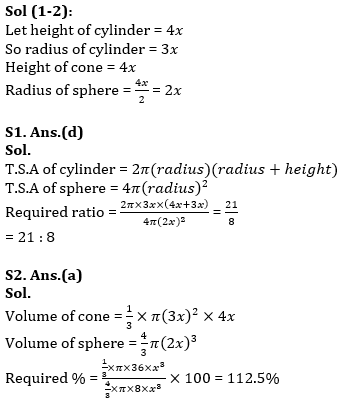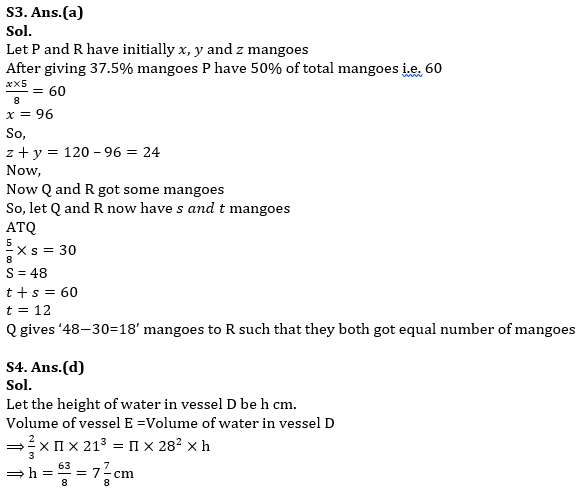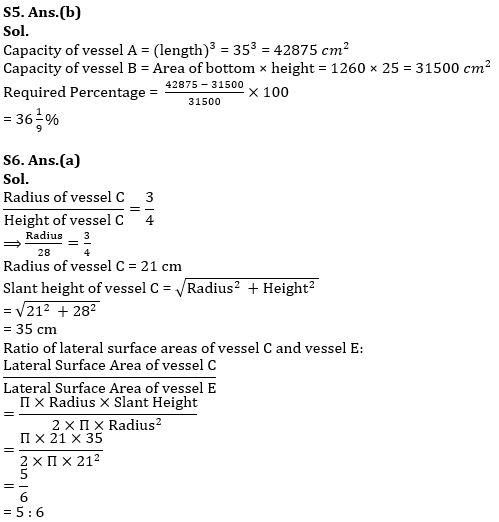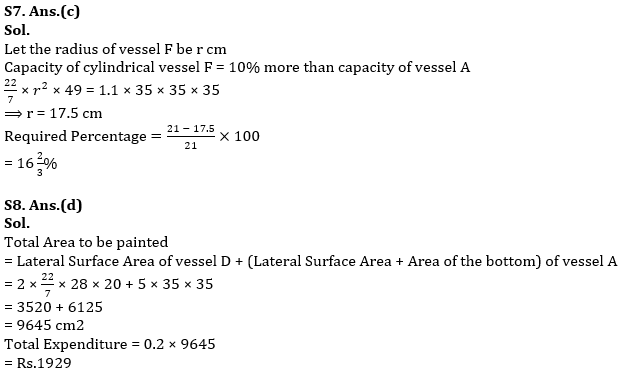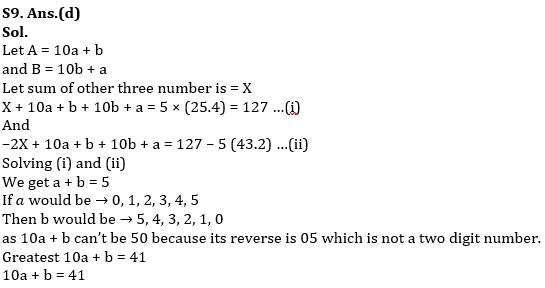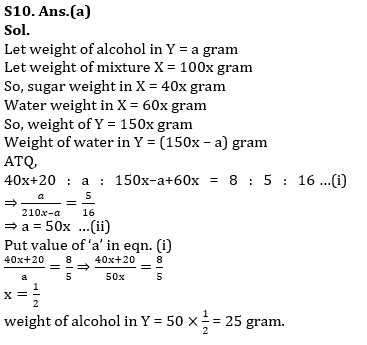#### Congratulations!